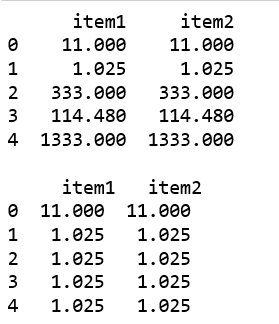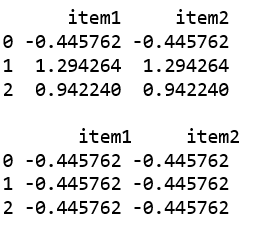# Python | Pandas Panel.cummin()

• Last Updated : 01 Jan, 2019

In Pandas, Panel is a very important container for three dimensional data. The names for the 3 axes are intended to give some semantic meaning to describing operations involving panel data and, in particular, econometric analysis of panel data.

`Panel.cummin()` function is used to returns a DataFrame or Series of the same size containing the cumulative minimum.

Attention geek! Strengthen your foundations with the Python Programming Foundation Course and learn the basics.

To begin with, your interview preparations Enhance your Data Structures concepts with the Python DS Course. And to begin with your Machine Learning Journey, join the Machine Learning - Basic Level Course

Syntax: Panel.cummin(axis=None, skipna=True, *args, **kwargs)

Parameters:
axis : The index or the name of the axis. 0 is equivalent to None or ‘index’.
skipna : Exclude NA/null values. If an entire row/column is NA, the result will be NA.

Returns: Cummin of DataFrame or Panel

Code #1:

 `# importing pandas module ``import` `pandas as pd ``import` `numpy as np`` ` `df1 ``=` `pd.DataFrame({``'a'``: [``'Geeks'``, ``'For'``, ``'geeks'``, ``'for'``, ``'real'``], ``                    ``'b'``: [``11``, ``1.025``, ``333``, ``114.48``, ``1333``]})``                     ` `data ``=` `{``'item1'``:df1, ``'item2'``:df1}`` ` `# creating Panel ``panel ``=` `pd.Panel.from_dict(data, orient ``=``'minor'``)`` ` `print``(panel[``'b'``])`` ` `print``(``"\n"``, panel[``'b'``].cummin(axis ``=` `0``))`

Output:Code #2:

 `# importing pandas module ``import` `pandas as pd ``import` `numpy as np`` ` `df1 ``=` `pd.DataFrame({``'a'``: [``'Geeks'``, ``'For'``, ``'geeks'``], ``                    ``'b'``: np.random.randn(``3``)})``                     ` `data ``=` `{``'item1'``:df1, ``'item2'``:df1}`` ` `# creating Panel ``panel ``=` `pd.Panel.from_dict(data, orient ``=``'minor'``)`` ` `print``(panel[``'b'``])`` ` ` ` `df2 ``=` `pd.DataFrame({``'b'``: [``11``, ``12``, ``13``]})``print``(``"\n"``, panel[``'b'``].cummin(axis ``=` `0``))`

Output:My Personal Notes arrow_drop_up